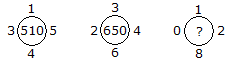# Verbal Reasoning - Character Puzzles - Discussion

### Discussion :: Character Puzzles - Character Puzzles 1 (Q.No.50)

50.

Which one will replace the question mark ?[A]. 660 [B]. 670 [C]. 610 [D]. 690

Explanation:

(1)2 + (5)2 + (4)2 + (3)2 = 51 x 10 = 510

and (3)2 + (4)2 + (6)2 + (2)2 = 65 x 10 = 650

Similarly (0)2 + (1)2 + (2)2 + (8)2 = 69 x 10 = 690.

 Gotham Ka Rakhwala said: (Mar 8, 2018) (1)^2 + (5)^2 + (4)^2 + (3)^2 = 510. How?

 Madhu said: (May 17, 2019) @Gotham. It's total 51 and multiplies with 10 = 510.